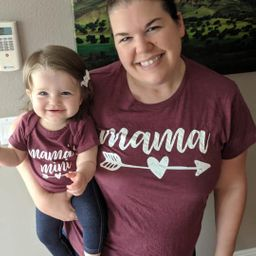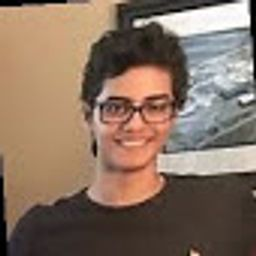# Geometry A Common Core Curriculum

## EducatorsProblem 1

WRITING Can a right triangle also be obtuse? Explain your reasoning.Ali S.

Problem 2

COMPLETE THE SENTENCE The of an exterior angle of a triangle is equal to the sum of the measures of the two _____ interior angles.Sally S.

Problem 3

In Exercises $3-6,$ classify the triangle by its sides and by measuring its angles.Ali S.

Problem 4

In Exercises $3-6,$ classify the triangle by its sides and by measuring its angles.Luca A.

Problem 5

In Exercises $3-6,$ classify the triangle by its sides and by measuring its angles.Ali S.

Problem 6

In Exercises $3-6,$ classify the triangle by its sides and by measuring its angles.Luca A.

Problem 7

In Exercises $7-10$ , classify $\triangle \mathrm{ABC}$ by its sides. Then determine whether it is a right triangle.
$$\mathrm{A}(2,3), \mathrm{B}(6,3), \mathrm{C}(2,7)$$Ali S.

Problem 8

In Exercises $7-10$ , classify $\triangle \mathrm{ABC}$ by its sides. Then determine whether it is a right triangle.
$$\mathrm{A}(3,3), \mathrm{B}(6,9), \mathrm{C}(6,-3)$$Luca A.

Problem 9

In Exercises $7-10$ , classify $\triangle \mathrm{ABC}$ by its sides. Then determine whether it is a right triangle.
$$\mathrm{A}(1,9), \mathrm{B}(4,8), \mathrm{C}(2,5)$$Ali S.

Problem 10

In Exercises $7-10$ , classify $\triangle \mathrm{ABC}$ by its sides. Then determine whether it is a right triangle.
$$\mathrm{A}(-2,3), \mathrm{B}(0,-3), \mathrm{C}(3,-2)$$Luca A.

Problem 11

In Exercises $11-14,$ $\mathrm{m} \angle 1 .$ Then classify the triangle by its angles.Ali S.

Problem 12

In Exercises $11-14,$ $\mathrm{m} \angle 1 .$ Then classify the triangle by its angles.Sally S.

Problem 13

In Exercises $11-14,$ $\mathrm{m} \angle 1 .$ Then classify the triangle by its angles.Ali S.

Problem 14

In Exercises $11-14,$ $\mathrm{m} \angle 1 .$ Then classify the triangle by its angles.Sally S.

Problem 15

In Exercises $15-18,$ nd the measure of the exterior angle.Ali S.

Problem 16

In Exercises $15-18,$ nd the measure of the exterior angle.Sally S.

Problem 17

In Exercises $15-18,$ nd the measure of the exterior angle.Ali S.

Problem 18

In Exercises $15-18,$ nd the measure of the exterior angle.Sally S.

Problem 19

In Exercises $19-22,$ I nd the measure of each acute angle.Ali S.

Problem 20

In Exercises $19-22,$ I nd the measure of each acute angle.Luca A.

Problem 21

In Exercises $19-22,$ I nd the measure of each acute angle.Ali S.

Problem 22

In Exercises $19-22,$ I nd the measure of each acute angle.Luca A.

Problem 23

In Exercises $23-26,$ ind the measure of each acute angle in the right triangle.
The measure of one acute angle is 5 times the measure of the other acute angle.Ali S.

Problem 24

In Exercises $23-26,$ ind the measure of each acute angle in the right triangle.
The measure of one acute angle is 8 times the measure of the other acute angle.Luca A.

Problem 25

In Exercises $23-26,$ ind the measure of each acute angle in the right triangle.
The measure of one acute angle is 3 times the sum of the measure of the other acute angle and $8 .$Ali S.

Problem 26

In Exercises $23-26,$ ind the measure of each acute angle in the right triangle.
The measure of one acute angle is twice the difference of the measure of the other acute angle and 12 .Luca A.

Problem 27

ERROR ANALYSIS In Exercises 27 and $28,$ describe and correct the error in 1 ending $\mathrm{m} \angle 1$Ali S.

Problem 28

ERROR ANALYSIS In Exercises 27 and $28,$ describe and correct the error in 1 ending $\mathrm{m} \angle 1$Luca A.

Problem 29

In Exercises $29-36,$ and the measure of the numbered angle.
$$\angle 1$$Ali S.

Problem 30

In Exercises $29-36,$ and the measure of the numbered angle.
$$\angle 2$$Luca A.

Problem 31

In Exercises $29-36,$ and the measure of the numbered angle.
$$\angle 3$$Ali S.

Problem 32

In Exercises $29-36,$ and the measure of the numbered angle.
$$\angle 4$$Luca A.

Problem 33

In Exercises $29-36,$ and the measure of the numbered angle.
$$\angle 5$$Ali S.

Problem 34

In Exercises $29-36,$ and the measure of the numbered angle.
$$\angle 6$$Luca A.

Problem 35

In Exercises $29-36,$ and the measure of the numbered angle.
$$\angle 7$$Ali S.

Problem 36

In Exercises $29-36,$ and the measure of the numbered angle.
$$\angle 8$$Luca A.

Problem 37

USING TOOLS Three people are standing on a stage. The distances between the three people are shown in the diagram. Classify the triangle by its sides and by measuring its angles.Ali S.

Problem 38

USING STRUCTURE Which of the following sets of angle measures could form a triangle? Select all
that apply.
A. $100^{\circ}, 50^{\circ}, 40^{\circ}$
B. $96^{\circ}, 74^{\circ}, 10^{\circ}$
C. $165^{\circ}, 113^{\circ}, 82^{\circ}$
D. $101^{\circ}, 41^{\circ}, 38^{\circ}$
E. $90^{\circ}, 45^{\circ}, 45^{\circ}$
F. $84^{\circ}, 62^{\circ}, 34^{\circ}$Luca A.

Problem 39

MODELING WITH MATHEMATICS You are bending a strip of metal into an isosceles triangle for a sculpture. The strip of metal is 20 inches long. The $|$ first bend is made 6 inches from one end. Describe two ways you could complete the triangle.Ali S.

Problem 40

THOUGHT PROVOKING Find and draw an object (or part of an object) that can be modeled by a triangle
and an exterior angle. Describe the relationship between the interior angles of the triangle and the
exterior angle in terms of the object.Luca A.

Problem 41

PROVING A COROLLARY Prove the Corollary to the Triangle Sum Theorem (Corollary 5.1$)$ .
Given $\triangle A B C$ is a right triangle. Prove $\angle$ A and $\angle B$ are complementary.Ali S.

Problem 42

PROVING A THEOREM Prove the Exterior Angle Theorem (Theorem 5.2$)$ .
Given $\triangle A B C$ , exterior $\angle B C D$ Prove $m \angle A+m \angle B=m \angle B C D$Luca A.

Problem 43

CRITICAL THINKING Is it possible to draw an obtuse isosceles triangle? obtuse equilateral triangle?
If so, provide examples. If not, explain why it is not possible.Ali S.

Problem 44

CRITICAL THINKING:Is it possible to draw a right isosceles triangle? right equilateral triangle?
If so, provide an example. If not, explain why it is not possible.Luca A.

Problem 45

MATHEMATICAL CONNECTIONS ABC is isosceles, $\mathrm{AB}=\mathrm{x},$ and $\mathrm{BC}=2 \mathrm{x}-4 .$
a. Find two possible values for $\mathrm{x}$ when the perimeter of $\Delta \mathrm{ABCis} 32$
b. How many possible values are there for $x$ when the perimeter of $\Delta$ ABC is 12$?$Ali S.

Problem 46

HOW DO YOU SEE IT In as many ways as possible, classify each triangle by its appearance.Luca A.

Problem 47

ANALYZING RELATIONSHIPS Which of the following could represent the measures of an exterior angle and two interior angles of a triangle? Select all that apply.
A. $100^{\circ}, 62^{\circ}, 38^{\circ}$
B. $81^{\circ}, 57^{\circ}, 24^{\circ}$
C. $119^{\circ}, 68^{\circ}, 49^{\circ}$
D. $95^{\circ}, 85^{\circ}, 28^{\circ}$
E. $92^{\circ}, 78^{\circ}, 68^{\circ}$
F. $149^{\circ}, 101^{\circ}, 48^{\circ}$Ali S.

Problem 48

MAKING AN ARGUMENT Your friend claims the measure of an exterior angle will always be
greater than the sum of the nonadjacent interior angle measures. Is your friend correct? ExplainLuca A.

Problem 49

MATHEMATICAL CONNECTIONS Exercises $49-52,$ I nd the values of $\mathrm{x}$ and $\mathrm{y}$ .Ali S.

Problem 50

MATHEMATICAL CONNECTIONS Exercises $49-52,$ I nd the values of $\mathrm{x}$ and $\mathrm{y}$ .Luca A.

Problem 51

MATHEMATICAL CONNECTIONS Exercises $49-52,$ I nd the values of $\mathrm{x}$ and $\mathrm{y}$ .Ali S.

Problem 52

MATHEMATICAL CONNECTIONS Exercises $49-52,$ I nd the values of $\mathrm{x}$ and $\mathrm{y}$ .Luca A.

Problem 53

PROVING A THEOREM Use the diagram to write a proof of the Triangle Sum Theorem (Theorem 5.1$)$ .
Your proof should be different from the proof of the Triangle Sum Theorem shown in this lesson.Ali S.

Problem 54

Use the diagram to 1 nd the measure of the segment or angle.
$$\mathrm{m} \angle \mathrm{KHL}$$

Check back soon!

Problem 55

Use the diagram to 1 nd the measure of the segment or angle.
$$\mathrm{m} \angle \mathrm{ABC}$$Ali S.

Problem 56

Use the diagram to 1 nd the measure of the segment or angle.
$$\mathrm{GH}$$

Check back soon!

Problem 57

Use the diagram to 1 nd the measure of the segment or angle.
$$\mathrm{BC}$$Ali S.